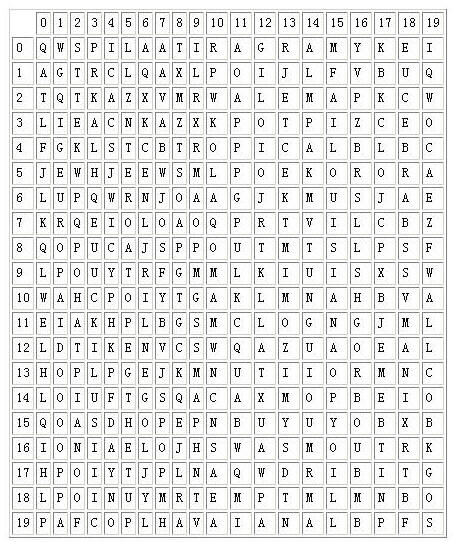# poj1204:AC自动机

Word Puzzles
 Time Limit: 5000MS Memory Limit: 65536K Total Submissions: 10848 Accepted: 4098 Special Judge

Description

Word puzzles are usually simple and very entertaining for all ages. They are so entertaining that Pizza-Hut company started using table covers with word puzzles printed on them, possibly with the intent to minimise their client's perception of any possible delay in bringing them their order.

Even though word puzzles may be entertaining to solve by hand, they may become boring when they get very large. Computers do not yet get bored in solving tasks, therefore we thought you could devise a program to speedup (hopefully!) solution finding in such puzzles.

The following figure illustrates the PizzaHut puzzle. The names of the pizzas to be found in the puzzle are: MARGARITA, ALEMA, BARBECUE, TROPICAL, SUPREMA, LOUISIANA, CHEESEHAM, EUROPA, HAVAIANA, CAMPONESA.Your task is to produce a program that given the word puzzle and words to be found in the puzzle, determines, for each word, the position of the first letter and its orientation in the puzzle.

You can assume that the left upper corner of the puzzle is the origin, (0,0). Furthemore, the orientation of the word is marked clockwise starting with letter A for north (note: there are 8 possible directions in total).

Input

The first line of input consists of three positive numbers, the number of lines, 0 < L <= 1000, the number of columns, 0 < C <= 1000, and the number of words to be found, 0 < W <= 1000. The following L input lines, each one of size C characters, contain the word puzzle. Then at last the W words are input one per line.

Output

Your program should output, for each word (using the same order as the words were input) a triplet defining the coordinates, line and column, where the first letter of the word appears, followed by a letter indicating the orientation of the word according to the rules define above. Each value in the triplet must be separated by one space only.

Sample Input

```20 20 10
QWSPILAATIRAGRAMYKEI
AGTRCLQAXLPOIJLFVBUQ
TQTKAZXVMRWALEMAPKCW
LIEACNKAZXKPOTPIZCEO
FGKLSTCBTROPICALBLBC
JEWHJEEWSMLPOEKORORA
LUPQWRNJOAAGJKMUSJAE
KRQEIOLOAOQPRTVILCBZ
QOPUCAJSPPOUTMTSLPSF
LPOUYTRFGMMLKIUISXSW
WAHCPOIYTGAKLMNAHBVA
EIAKHPLBGSMCLOGNGJML
LDTIKENVCSWQAZUAOEAL
HOPLPGEJKMNUTIIORMNC
LOIUFTGSQACAXMOPBEIO
IONIAELOJHSWASMOUTRK
HPOIYTJPLNAQWDRIBITG
LPOINUYMRTEMPTMLMNBO
PAFCOPLHAVAIANALBPFS
MARGARITA
ALEMA
BARBECUE
TROPICAL
SUPREMA
LOUISIANA
CHEESEHAM
EUROPA
HAVAIANA
CAMPONESA```

Sample Output

```0 15 G
2 11 C
7 18 A
4 8 C
16 13 B
4 15 E
10 3 D
5 1 E
19 7 C
11 11 H```

Source

AC自动机。先用给出的单词构造AC自动机，然后在字符矩阵里提取字符串进行匹配。我的提取方法是：只提取字符矩阵边缘上和各条斜线的八个方向上的字符串（为什么要说“各条”？因为矩阵并不总是一个正方形矩阵），这样就能节省时间了。

``````
/*Source Code
Problem: 1204		User: aclolicon
Memory: 13048K		Time: 1094MS
Language: C++		Result: Accepted
Source Code*/
#include<cstdio>
#include<queue>
#include<cstring>
#define MAXN 1080
#define MAXNODE 600000
#define RANGE 26
using namespace std;
int node[MAXNODE][RANGE];
bool val[MAXNODE];
int nc = 1;//Node counting
char p[MAXN][MAXN];
int f[MAXNODE];
bool chkword[MAXNODE];
char direction = {{'H', 'A', 'B'}, {'G', ' ', 'C'}, {'F', 'E', 'D'}};
int last[MAXNODE];
int getwo[MAXNODE];
char tmp[MAXN];
char ansd[MAXN];
int anspos[MAXN];
int row, col, wc;
char word[MAXN][MAXN], tc;
int indx(char c){
return c - 'A';
}
void getans(int pos, int n, int x, int y, int d1, int d2){
if (val[n]) {
int length = strlen(word[getwo[n]]);
pos -= length - 1;
ansd[getwo[n]] = tc;
anspos[getwo[n]] = x + pos * d1;
anspos[getwo[n]] = y + pos * d2;
}
}
void findt(char* t, int x, int y, int d1, int d2){
int len = strlen(tmp);
if (len == 0) return;
int j = 0;
for (int i = 0; i < len; i++){
int c = indx(t[i]);
while(j && !node[j][c]) j = f[j];
j = node[j][c];
if (val[j]) {
getans(i, j, x, y, d1, d2);
getans(i, last[j], x, y, d1, d2);
}
else if (last[j]){
getans(i, last[j], x, y, d1, d2);
}
}
}
void find(int x, int y){
int cnt, tx = x, ty = y;
for (int i = -1; i < 2; i++)
for (int j = -1; j < 2; j++){
cnt = 0;
memset(tmp, 0, sizeof(tmp));
if (i == 0 && j == 0) continue;
tx = 0, ty = 0;
while(1){
if (tx < 0 || ty < 0 || tx >= row || ty >= col) break;
tx = x + i * cnt, ty = y + j * cnt;
tmp[cnt++] = p[tx][ty];
}
tc = direction[1 + i][1 + j];
findt(tmp, x, y, i, j);
}
}
void getfail(){
int u, r, v;
queue<int> q;
for (int i = 0; i < RANGE; i++){
u = node[i];
if (u){
f[u] = 0;
q.push(u);
last[u] = 0;
}
}
while(!q.empty()){
r = q.front();
q.pop();
for (int i = 0; i < RANGE; i++){
u = node[r][i];
if (!u) {
node[r][i] = node[f[r]][i];
continue;
}
q.push(u);
v = f[r];
f[u] = node[v][i];
last[u] = val[f[u]] ? f[u] : last[f[u]];
}
}
}
int len = strlen(w);
int t = 0;
for (int i = 0; i < len; i++){
int idx = indx(w[i]);
if (!node[t][idx]){ //没有相应单词结点
val[nc] = 0;
node[t][idx] = nc++;
}
t = node[t][idx];
}
val[t] = 1;
getwo[t] = num;
}
int main(){
//	freopen("1204.in", "r", stdin);
//	freopen("c.out", "w", stdout);
scanf("%d%d%d", &row, &col, &wc);
for (int i = 0; i < row; i++)
scanf("%s", p[i]);
for (int i = 0; i < wc; i++){
scanf("%s", word[i]);
}
getfail();
for (int i = 0; i < row; i++) find (i, col - 1);
for (int j = 0; j < col; j++) find (row - 1, j);
for (int i = 0; i < row; i++) find (i, 0);
for (int j = 0; j < col; j++) find (0, j);
for (int i = 0; i < wc; i++){
printf("%d %d %c\n", anspos[i], anspos[i], ansd[i]);
}
return 0;
}
``````## 6 条评论

1.薛之谦

666666

•a/b

活捉大触Σ(･ω･ﾉ)ﾉ

•薛之谦

Σ(･ω･ﾉ)ﾉ你才是大触

2.小易分享网

谢谢博主分享，很实用！ http://www.xevip.cn

3.奇虎分享网

哎呦，不错哦！欢迎互访，诚交友链！

4.VIP灵儿网

这个文章写的好，转走了！ 欢迎回访:http://www.viplinger.cn